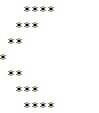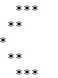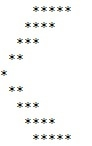# C Program for Arrow Star Pattern

Given a number n and we have to print the arrow star pattern of maximum n number of stars.

The star pattern of input 4 will look like −## Example

Input: 3
Output:Input: 5
Output:The approach used below is as follows −

• Take input in integer.
• Then print n spaces and n stars.
• Decrement till n>1.
• Now increment till n.
• And print spaces and stars in increasing order.

## Algorithm

Start
In function int arrow(int num)
Step 1-> declare and initialize i, j
Step 2-> Loop For i = 1 and i <= num and i++
Loop For j = i and j < num and j++
Print a space
Loop For j = i and j <= num and j++
Print "*"
Print newline
Step 3-> Loop For i = 2 and i <= num and i++
Loop For j= 1 and j < I and j++
Print a space
Loop For j = 1 and j <= i and j++
Print "*"
Print newline
In function int main()
Step 1-> declare and initialize num = 4
Step 2-> call arrow(num)

## Example

Live Demo

#include <stdio.h>
// arrow function
int arrow(int num) {
int i, j;
// Prints the upper part of the arrow
for (i = 1; i <= num; i++) {
// to print the spaces
for (j = i; j < num; j++) {
printf(" ");
}
// to print the * for the pattern
for (j = i; j <= num; j++) {
printf("*");
}
printf("");
}
// Prints lower part of the arrow
for (i = 2; i <= num; i++) {
// to print the spaces
for (j = 1; j < i; j++) {
printf(" ");
}
// to print the * for the pattern
for (j = 1; j <= i; j++) {
printf("*");
}
printf("");
}
return 0;
}
int main() {
// get the value from user
int num = 4;
// function calling
arrow(num);
return 0;
}

## Output

If run the above code it will generate the following output −